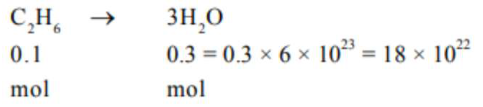# Complete combustion of 3 g of ethane gives x .Question:

Complete combustion of $3 \mathrm{~g}$ of ethane gives $\mathrm{x} \times 10^{22}$ molecules of water.

The value of $x$ is ______________ .(Round off to the Nearest Integer).

$\left[\right.$ Use $: \mathrm{N}_{\mathrm{A}}=6.023 \times 10^{23} ;$ Atomic masses in $\mathrm{u}$$: C: 12.0 ; O: 16.0 ; H: 1.0]$

Solution:

(18)No. of molecules $=0.3 \times 6.023 \times 10^{23}$

$=18.069 \times 10^{22}$